# 643 and Level 1

Here’s an interesting prime factorization: 2⁶⋅3⋅643 = 123456. Did you notice its largest prime factor? Thank you OEIS.org for that fun fact about the number 643.Print the puzzles or type the solution on this excel file: 10 Factors 2015-10-12

—————————————————————————————————

• 643 is a prime number. 641 and 643 are twin primes.
• Prime factorization: 643 is prime.
• The exponent of prime number 643 is 1. Adding 1 to that exponent we get (1 + 1) = 2. Therefore 643 has exactly 2 factors.
• Factors of 643: 1, 643
• Factor pairs: 643 = 1 x 643
• 643 has no square factors that allow its square root to be simplified. √643 ≈ 25.357444666.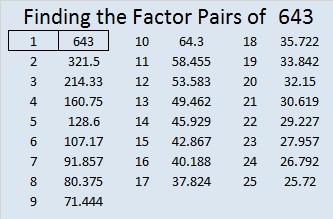How do we know that 643 is a prime number? If 643 were not a prime number, then it would be divisible by at least one prime number less than or equal to √643 ≈ 25.4. Since 643 cannot be divided evenly by 2, 3, 5, 7, 11, 13, 17, 19, or 23, we know that 643 is a prime number.

—————————————————————————————————# 21 Factors of the Year 2013 and 2014

21 is a composite number. 21 = 1 x 21 or 3 x 7. Factors of 21: 1, 3, 7, 21. Prime factorization: 21 = 3 x 7.When 21 is a clue in the FIND THE FACTORS puzzles, use 3 x 7.

Scroll down the page to find factoring information about 2013 and 2014.Near the end of each year movie critics make lists of the ten best movies and the ten worse movies of the year. News agencies list the ten most significant news stories. Time magazine lists the ten most influential people of the year. The music industry lists the top ten songs of the year. As 2013 draws to a close, it is most appropriate for me to review the factors of the year.

2013 had exactly 8 positive factors. These factors were 1, 3, 11, 33, 61, 183, 671, and 2013.

There is no room for argument. I am absolutely certain this list is complete. No one will make any comments disagreeing with me, calling me names, or asking how I could have left Two or Five or Seven off the list. Also no one will wonder why I would include forgettable 671 on the list. Do the Math. 671 was clearly a factor in 2013. Three of the factors of 2013 were also prime factors. They were 3, 11, and 61. This graphic clearly shows those prime factors.2013 also had 8 negative factors. The first negative factor on the list is no surprise: Minus One. Year in and year out we can count on Minus One being a negative factor. Some other factors were just as negative in 2013, namely -3, -11, -33, – 61, -183, -671, and -2013. Of course, many of those factors were so obscure that most people never gave them a second thought all year long. Again I expect no arguments or negative comments on these selections. Anyone who knows anything about factors will have to agree with this list.

Even though 2014 hasn’t even started, I am going to predict the factors of 2014, and I am absolutely positive that my predictions will be 100% correct. You will not even have to wait until the end of 2014 to verify my accuracy.

The positive factors of 2014 will be (drum roll) 1, 2, 19, 38, 53, 106,1007, and 2014.

Most people expect the number One to be a positive factor every single year, and it will not let us down in 2014. The number Two has a reputation of being a factor only about half the time. Since she was not a factor at all in 2013, I am confident that she will get her act together again in 2014 and become a factor once more. All the other factors I’ve listed have not been factors for a very long time, and each one of them is due to make a difference over and over again in 2014 until they have nothing leftover. I predict that 2014 will have three prime factors, namely 2, 19, and 53, as illustrated in the following graphic.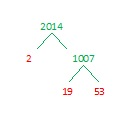How can I make such accurate assessments and spot on predictions? I will tell you: I work with factors almost every single day, and I’ve spent years observing them. Every time I have been given an assignment to become acquainted with them, I have approached that assignment with enthusiasm and determination.

Regardless of my astounding record, YOU can become just as much an expert as I am with just a little bit of knowledge and effort. You may discover, as I have, that factoring can be great fun. Here are a couple of logic puzzles that require factoring to solve:

All you have to do to solve one of the puzzles is write the numbers 1 – 12 in the top row and again in the first column so that those numbers are the factors of the given clues. Each puzzle has only one solution.

At the top of this post is a page titled How to Find the Factors, and it gives hints to solve the puzzles.   Click 12 Factors 2013-12-30 to find a printable version of these and a few other puzzles as well as the solutions for last week’s puzzles. Excel or comparable spreadsheet program is needed to open the file.

Have a great 2014 and happy factoring!

Related Articles

# 15 is the Magic Sum of a 3 x 3 Magic Square

15 is a composite number. 15 = 1 x 15 or 3 x 5. Factors of 15: 1, 3, 5, 15. Prime factorization: 15 = 3 x 5.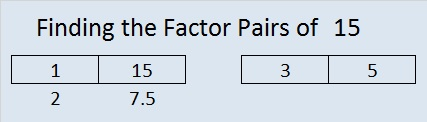When 15 is a clue in the FIND THE FACTORS 1 – 10 or 1 – 12 puzzles, use 3 and 5 as the factors.

If you added the first nine counting numbers together, what sum would you get? What is 1 + 2 +3 + 4+ 5 + 6 + 7 + 8 + 9?

Would you get the same answer by adding (1 + 9) + (2 + 8) + (3 +7) + (4 + 6) + 5?

These are two of the many fun questions you can explore when you try to make a magic square. What is a magic square? If you can place the numbers from 1 to 9 in the box below so that the sum of any row, column, or diagonal will equal the sum of any other row, column, or diagonal, then you will have made a 3 x 3 magic square. The sum of a row, column, or diagonal in a magic square is called the magic sum.Clearly it is not a magic square yet. In fact, only one of the numbers is positioned where it needs to be. Which number do you think is already in the correct position?

When it becomes a magic square, what will the magic sum be? One student noticed that in its current state the sums of the rows are 6, 15, and 24. The sums of the columns are 12, 15, 18. The sums of the diagonals are 15 and 15. Since 15 occurs most often, could the magic sum be 15? One way to determine what the magic sum should be is to add the sums of all three rows and then divide by the number of rows. Since 1 + 2 + 3 + 4 + 5 + 6 + 7 + 8 + 9 = 45 and 45 ÷ 3 = 15, then 15 is indeed the magic sum.

Here are a few easy-to-remember steps to construct a 3 x 3 magic square quickly.

Step 1: Draw a tic-tac-toe board and put 5 in the middle.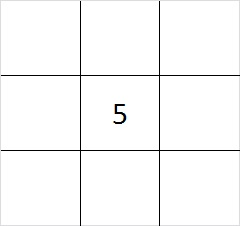Step 2: Put one of the even numbers in one of the corners.  You have four different choices, 2, 4, 6, or 8. The illustration is for the number 2, but any of the even numbers will work.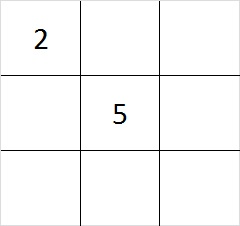Step 3: Subtract your even number from 10 to find its partner. 4 + 6 are partners and so are 2 + 8. Put the partner of the number you chose for step 1 in the corner that is diagonal to it.Step 4: Put the other two even numbers in the remaining corners. Yes, you have two choices where to put the numbers. Either choice will work.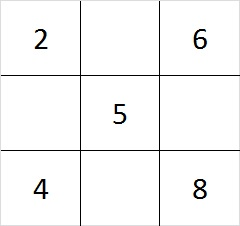Step 5: Since 6 + 8 = 14 and 15 – 14 = 1, put 1 in the cell between the 6 and the 8. Do similar addition and subtraction problems on each side of the square to determine where to place the 3, 7, and 9. You can work clockwise or counter clockwise, or skip around the square doing the addition and subtraction problems; it doesn’t matter.

This finished magic square looks like this: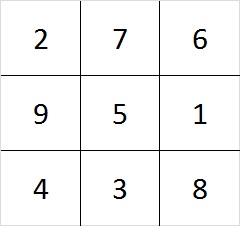Check it out! Every row, column, and diagonal adds up to 15!

As we created the square, we made choices. First we chose between 4 even numbers, and later we had 2 more choices. Notice that 4 x 2 = 8. There are 8 different ways to make a 3 x 3 magic square! (However, they are all really the same square turned upside down, rolled on its side, viewed from the back. etc.)

There are 880 different ways to make a 4 x 4 magic square. Look over the related articles at the end of this post to learn more about magic squares that are bigger than 3 x 3.

Speaking of magic squares, when I look at the square logic puzzle below, something magical happens. This puzzle has nine clues in it, and all of them are perfect squares. I can use those nine clues to construct a complete multiplication table. If you finish the same puzzle, your multiplication table will look exactly like mine because this puzzle has only one solution.The level 3 puzzle below is only a little bit more difficult. To solve it place the numbers 1 – 10 in the top row and again in the first column so that those placed numbers are the factors of the given clues. Again there is only one solution, and you will need to use logic to find it. Click 10 Factors 2014-01-06 for more puzzles and last week’s answers.May we all find a little bit more magic in our lives!

# 6 A Piece of Cake

### Birthday Puzzles for My Daughter:

Happy birthday, Kathy! I hope your day is wonderful. You have grown into a beautiful, talented, prayerful, intelligent, hard-working, and loving young woman.  I am grateful you are my daughter.  So for your birthday today and for this blog, I’ve created three special puzzles: the first is a birthday cake to celebrate your happy day. To highlight your love of music, the second puzzle is a quarter note. The third puzzle is either a violin, a guitar, or a ukulele, you decide. I love listening as you sing or as you play any of those instruments or the piano. Today for your birthday I will also cut down a tree and make yet another cake with two birthday candles on top in this blog post.  So have a fun birthday, today.  I love you.Click 12 Factors 2013-11-14 for more puzzles.### Factor Trees vs. Factor Cakes:

What did I mean by cutting down a tree and making yet another cake? Today I will discuss two methods for finding the prime factors of a whole number. One method is making a factor tree and the other is the cake method. To factor a number means to write it as the product of two or more factors. When those two or more factors are all prime factors, it is called a prime factorization of the number. A composite number always has more than two factors. A prime number always has exactly 2 factors, 1 and itself. (ZERO and ONE are neither prime or composite numbers.) Usually, to find the prime factors of a number, a person will usually make a factor tree. The following example shows how this is done:From this example, you can certainly understand why this algorithm is called a factor tree.  It looks exactly like a perfectly-shaped evergreen tree.  The problem is that a factor tree doesn’t always look so neat and trim.  Here is a factor tree that even Charlie Brown wouldn’t choose:720 isn’t even that big of a number, but gathering all of the prime numbers from the factor tree and putting them in numerical order would be like picking up a bunch of scattered leaves. It would be like doing . . . yard work.  Imagine if you had a number that had many more factors. If one or two of the factors gets lost in the mess, your answer wouldn’t be correct. Notice that some of the prime factors of 720 (2,2,2,2,3,3,5) are not as easy to see as others on the factor tree.  That is why I want to chop down that tree. Even if you like to do yard work, do you really want to deal with that big of a mess, . . . especially when you can have cake instead?  Look, the cake method is so much more pleasing to the eye, and it is simply an extension of the very familiar division algorithm:With the cake method, the more factors you have, the bigger the cake will be, but it will always be neatly organized with all the factors on the outside of the cake.  And if the largest prime factor of your given number is eleven, you will also have two candles on top of your cake!  I find using the cake method to be much less confusing than using a factor tree.  Yes, finding prime factors can actually be a piece of cake. The only disadvantage to the cake method is that since you work from the bottom up you have to leave enough space for the cake to rise.

Still, in spite of my opinion, it is best to use whichever method you are more comfortable with.

Now if your appetite for cake has not been satisfied, click on one of the links below for a nice variety of cakes shared by other bloggers.

### Factors of the Number 6:6 is a composite number. 6 = 1 x 6 or 2 x 3. Factors of 6: 1, 2, 3, 6. Prime factorization: 2 x 3.When 6 is a clue in the FIND THE FACTORS  puzzle, the pair that will work for that particular puzzle might be 1 x 6, or it might be 2 x 3.

### A Sum-Difference Puzzle Featuring the Number 6 and its Factors:

Look at the factor pair puzzle above. Perhaps you will notice that
2 + 3 = 5 and 6 – 1 = 5.
Those are the facts you need to complete the Sum-Difference puzzle below.